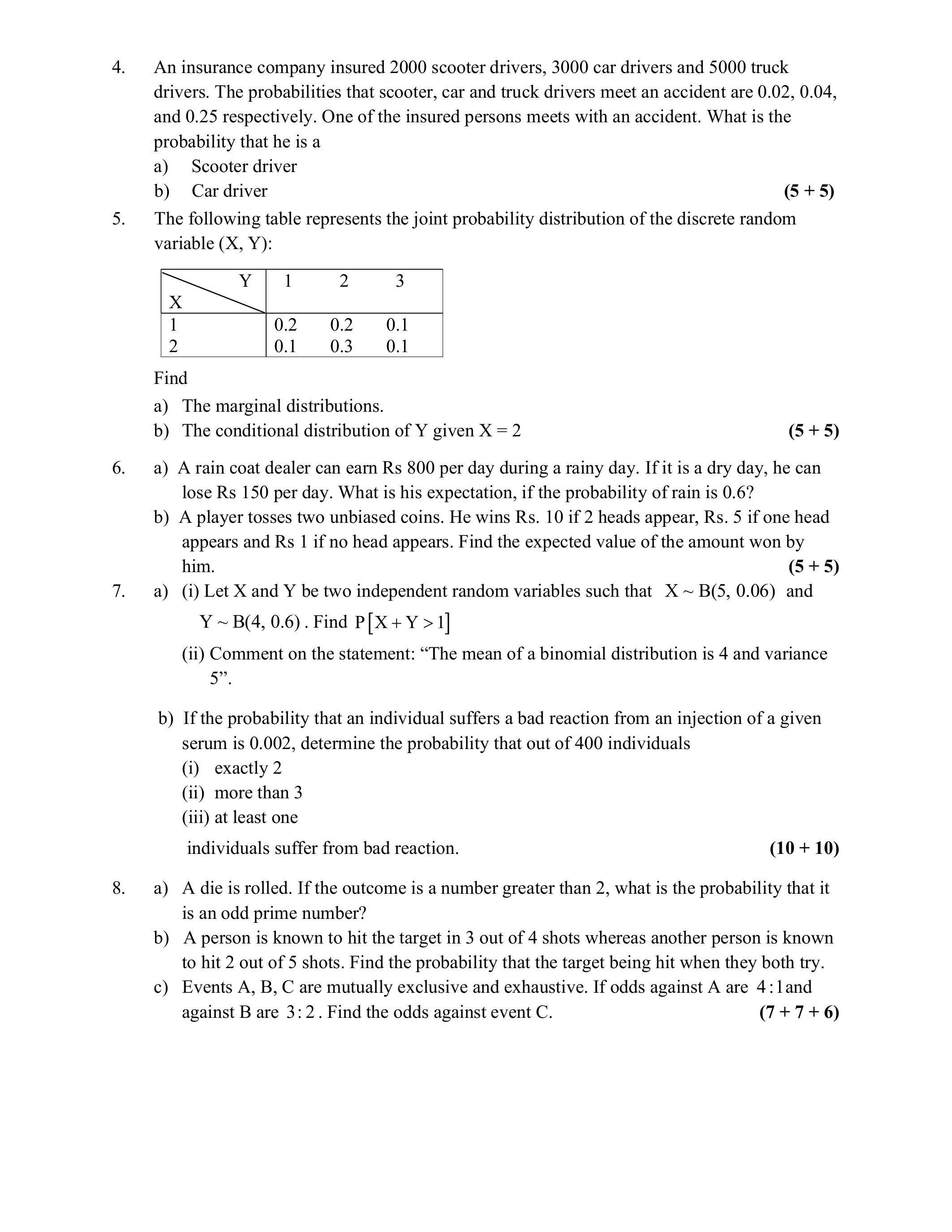# IGNOU MST-003 Solved Assignment 2023 | PGDAST

Details For MST-003 Solved Assignment

## IGNOU MST-003 Assignment Question Paper 2023

Get ahead in your IGNOU PGDAST Course studies with confidence by reviewing the MST-003 assignment questions before buying the expert-written solution from our app. Quick Search Section in our app makes it easy to double-check the year and session validity of the IGNOU assignment solutions, ensuring that you avoid any confusion. Our aim is to provide you with the most accurate and up-to-date IGNOU assignment solutions for PGDAST, and our expert-written assignments are unmatched by any other website. So, before making your purchase, take a moment to review the MST-003 assignment questions and ensure they match your needs. With our attention to detail and expertise, you can trust that you’re getting the right IGNOU assignment solution for your specific needs.

1. Which of the following statements are true or false? Give reason in support of your answer.

a) When two dice are thrown simultaneously then total number of sample points in the sample space will be 12 .

b) Expected value of a continuous random variable $$X$$ is defined as $$E(X)=\int_{-\infty}^{x} x f(x) d x$$.

c) If $$X$$ and $$Y$$ are independent random variable then $$\mathrm{V}(\mathrm{X}-\mathrm{Y})=\mathrm{V}(\mathrm{X})-\mathrm{V}(\mathrm{Y})$$.

d) If $$X \sim B(4,3)$$ then variance of $$X$$ is 12 .

e) If probability density function of a normally distributed random variable $$\mathrm{X}$$ is

$f(x)=\frac{1}{6 \sqrt{2 \pi}} e^{-\frac{1}{2}\left(\frac{x-46}{6}\right)^{2}}, \quad-\infty<x<\infty$

then variance of $$\mathrm{X}$$ is 36 .

2. An insurance company selected 6000 drivers from a city at random in order to find a relationship between age and accidents. The following table shows the results to these 6000 drivers.

 Age of drivers (in years) Class Interval Accidents in one year 0 1 2 3 4 or more 18−25 700 310 225 110 85 25−40 1100 290 200 105 80 40−50 1200 235 175 80 55 50 and above 600 205 140 70 35

If a driver from the city is selected at random, find the probability of the following events:

a) Age lying between $$18-25$$ and meet 3 accidents

b) Age lying between $$18-40$$ and meet 1 accident

c) Age more than 25 years and meet at most one accident

d) Having no accident in the year

e) Age lying between $$18-40$$ and meet at least 3 accidents

3. Determine the constant $$\mathrm{k}$$ such that the function $$\mathrm{f}(\mathrm{x})=\mathrm{kx}^{2}(1-\mathrm{x})^{5}, 0<\mathrm{x}<1$$ is a beta distribution of first kind. Also, find its mean and variance.

4. An insurance company insured 2000 scooter drivers, 3000 car drivers and 5000 truck drivers. The probabilities that scooter, car and truck drivers meet an accident are 0.02, 0.04, and $$0.25$$ respectively. One of the insured persons meets with an accident. What is the probability that he is a

a) Scooter driver

b) Car driver

5. The following table represents the joint probability distribution of the discrete random variable $$(\mathrm{X}, \mathrm{Y})$$ :Find

a) The marginal distributions.

b) The conditional distribution of $$Y$$ given $$X=2$$

6. a) A rain coat dealer can earn Rs 800 per day during a rainy day. If it is a dry day, he can lose Rs 150 per day. What is his expectation, if the probability of rain is $$0.6 ?$$

b) A player tosses two unbiased coins. He wins Rs. 10 if 2 heads appear, Rs. 5 if one head appears and Rs 1 if no head appears. Find the expected value of the amount won by him.

7. a) (i) Let $$\mathrm{X}$$ and $$\mathrm{Y}$$ be two independent random variables such that $$\mathrm{X} \sim \mathrm{B}(5,0.06)$$ and $$\mathrm{Y} \sim \mathrm{B}(4,0.6)$$. Find $$\mathrm{P}[\mathrm{X}+\mathrm{Y}>1]$$

(ii) Comment on the statement: “The mean of a binomial distribution is 4 and variance 5”.

b) If the probability that an individual suffers a bad reaction from an injection of a given serum is $$0.002$$, determine the probability that out of 400 individuals

(i) exactly 2

(ii) more than 3

(iii) at least one individuals suffer from bad reaction.

8. a) A die is rolled. If the outcome is a number greater than 2 , what is the probability that it is an odd prime number?

b) A person is known to hit the target in 3 out of 4 shots whereas another person is known to hit 2 out of 5 shots. Find the probability that the target being hit when they both try.

c) Events A, B, C are mutually exclusive and exhaustive. If odds against A are $$4: 1$$ and against $$\mathrm{B}$$ are $$3: 2$$. Find the odds against event $$\mathrm{C}$$.

$$\frac{a}{sin\:A}=\frac{b}{sin\:B}=\frac{c}{sin\:C}$$

## MST-003 Sample Solution 2023

Take a look at our sample solution of MST-003 Solved Assignment 2023 | PGDAST to get a feel for the top notch and accuracy of our IGNOU assignment solution. This sample solution is just one example of how we can help you with your IGNOU assignments, and we’re happy to present it as proof that we always produce high-quality work done by subject matter experts. Our assignments are solved by experts from multiple universities, unlike other sites that provide copied or stolen study materials. By doing so, we can guarantee that you will receive solutions that are both original and accurate, providing you with the knowledge you need to succeed academically. Don’t take our word for it; have a look at a sample of our MST-003 Solved Assignment 2023 | PGDAST now and see for yourself. You’ll have the advantage you need to excel in your IGNOU studies with our expert-written assignments.

1(a)$$cos\left(2\theta \right)=cos^2\theta -sin^2\theta$$

## Price - 351 Rs

Insert math as
$${}$$